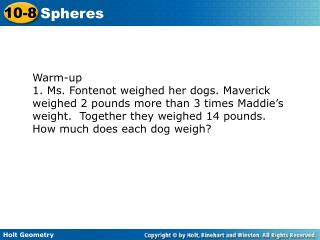# Warm-up - PowerPoint PPT PresentationDownload PresentationWarm-up

Warm-upDownload Presentation## Warm-up

- - - - - - - - - - - - - - - - - - - - - - - - - - - E N D - - - - - - - - - - - - - - - - - - - - - - - - - - -
##### Presentation Transcript

1. Warm-up 1. Ms. Fontenot weighed her dogs. Maverick weighed 2 pounds more than 3 times Maddie’s weight. Together they weighed 14 pounds. How much does each dog weigh?

2. A sphere is the locus of points in space that are a fixed distance from a given point called the center of a sphere. A radius of a sphere connects the center of the sphere to any point on the sphere. A hemisphere is half of a sphere. A great circle divides a sphere into two hemispheres

3. Example 1A: Finding Volumes of Spheres Find the volume of the sphere. Give your answer in terms of . Volume of a sphere. 12 = 2304 in3 Simplify.

4. Example 1B: Finding Volumes of Spheres Find the diameter of a sphere with volume 36,000 cm3. Volume of a sphere. Substitute 36,000 for V. 27,000 = r3 r = 30 Take the cube root of both sides. d = 60 cm d = 2r

5. Example 1C: Finding Volumes of Spheres Find the volume of the hemisphere. Volume of a hemisphere Substitute 15 for r. = 2250 m3 Simplify.

6. Check It Out! Example 1 Find the radius of a sphere with volume 2304ft3. Volume of a sphere Substitute for V. r = 12 ft Simplify.

7. Example 3A: Finding Surface Area of Spheres Find the surface area of a sphere with diameter 76 cm. Give your answers in terms of . S = 4r2 Surface area of a sphere S = 4(38)2 = 5776 cm2

8. Example 3B: Finding Surface Area of Spheres Find the volume of a sphere with surface area 324 in2. Give your answers in terms of . S = 4r2 Surface area of a sphere Substitute 324 for S. 324 = 4r2 r = 9 Solve for r. Substitute 9 for r. The volume of the sphere is 972 in2.

9. Example 3C: Finding Surface Area of Spheres Find the surface area of a sphere with a great circle that has an area of 49 mi2. A = r2 Area of a circle Substitute 49 for A. 49 = r2 r = 7 Solve for r. S = 4r2 = 4(7)2 = 196 mi2 Substitute 7 for r.

10. Check It Out! Example 3 Find the surface area of the sphere. S = 4r2 Surface area of a sphere S = 4(25)2 Substitute 25 for r. S = 2500 cm2

11. radius multiplied by : Notice that . If the radius is multiplied by , the volume is multiplied by , or . Example 4: Exploring Effects of Changing Dimensions The radius of the sphere is multiplied by . Describe the effect on the volume. original dimensions:

12. Check It Out! Example 4 The radius of the sphere is divided by 3. Describe the effect on the surface area. original dimensions: dimensions divided by 3: S = 4r2 S = 4r2 = 4(3)2 = 36 m3 = 4(1)2 = 4 m3 The surface area is divided by 9.

13. Example 5: Finding Surface Areas and Volumes of Composite Figures Find the surface area and volume of the composite figure. Give your answer in terms of . Step 1 Find the surface area of the composite figure. The surface area of the composite figure is the sum of the curved surface area of the hemisphere, the lateral area of the cylinder, and the base area of the cylinder.

14. Example 5 Continued Find the surface area and volume of the composite figure. Give your answer in terms of . L(cylinder) = 2rh = 2(6)(9) = 108 in2 B(cylinder) = r2 = (6)2 = 36 in2 The surface area of the composite figure is 72 + 108 + 36 = 216 in2.

15. Example 5 Continued Find the surface area and volume of the composite figure. Give your answer in terms of . Step 2 Find the volume of the composite figure. The volume of the composite figure is the sum of the volume of the hemisphere and the volume of the cylinder. The volume of the composite figure is 144 + 324 = 468 in3.